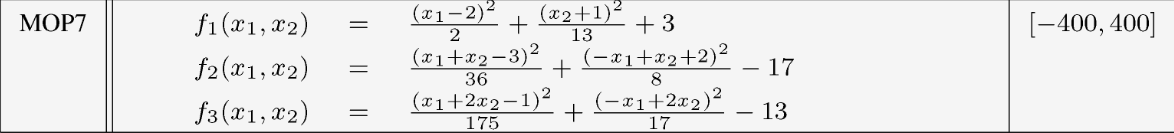## NSGAII.jl

A NSGA-II implementation in Julia
Author gsoleilhac
Popularity
17 Stars
Updated Last
1 Year Ago
Started In
October 2017

# References

A Fast Elitist Non-Dominated Sorting Genetic Algorithm for Multi-Objective Optimization: NSGA-II Kalyanmoy Deb, Samir Agrawal, Amrit Pratap, and T Meyarivan

# Installation

``````julia> ]
``````

# Usage

## Example : Bi-Objective Knapsack

```using NSGAII
n = 20 #Number of items
p1 = [77,94,71,63,96,82,85,75,72,91,99,63,84,87,79,94,90,60,69,62] #Coeffs objective 1
p2 = [65,90,90,77,95,84,70,94,66,92,74,97,60,60,65,97,93,60,69,74] #Coeffs objective 2
w = [80,87,68,72,66,77,99,85,70,93,98,72,100,89,67,86,91,79,71,99] #Items weights
c = 900 #Knapsack capacity```

The four mandatory parameters of NSGAII are

• the size of the population
• the number of generations
• an initialization function
• an evaluation function
```using Random: bitrand
using LinearAlgebra: dot

popsize = 100
nbgen = 200
init() = bitrand(n) #our genotype is a binary vector of size n, initialized randomly
z(x) = dot(x, p1), dot(x, p2) #and our objectives are the sum of the items we pick```

Now, this would be enough to run nsga-2 with `nsga_max(popsize, nbgen, z, init)`
But we need to add the constraint that all items must fit in the knapsack.
For this we define a constraint-violation function that returns 0 only if the solution is feasible,
and a value > 0 otherwise.

```function CV(x)
sumW = dot(x, w)
return sumW <= c ? 0 : sumW - c
end

#We can now call
result = nsga_max(popsize, nbgen, z, init, fCV = CV)```

`result` will be a vector of individuals.
The revelant fields of an individual `indiv` are :

• genotype : `indiv.x`
• objective values : `indiv.y`
• rank : `indiv.rank`
• constraint violation value : `indiv.CV`

### Crossover

If the solutions are encoded as bitstrings, a 2-point crossover will be used by default, but we can define our own and assign it with the keyword `fcross`:

```function one_point_crossover!(parent_a, parent_b, child_a, child_b)
n = length(parent_a)
cut = rand(1:n-1)

child_a[1:cut] .= parent_a[1:cut]
child_a[cut+1:n] .= parent_b[cut+1:n]

child_b[1:cut] .= parent_b[1:cut]
child_b[cut+1:n] .= parent_a[cut+1:n]
end

nsga_max(popsize, nbgen, z, init, fCV = CV, fcross = one_point_crossover!)```

For permutations genotypes, the default crossover is the PMX (Partially-Mapped Crossover)

### Mutation

The default mutation for a binary vector is the bitstring mutation where each bit has a probability 1/l to be flipped (where l is the length of the vector)

As with crossovers, we can define or own mutation operator and assign it with the keyword `fmut`. The probability of mutation can be changed with the keyword `pmut`.

Let's say we want our mutation to flip two random bits :

```function two_bits_flip!(bits)
for i = 1:2
n = rand(1:length(bits))
bits[n] = 1 - bits[n]
end
end

nsga_max(popsize, nbgen, z, init, fCV = CV, fmut = two_bits_flip!, pmut = 0.2)```

For permutations genotypes, the default mutation randomly swaps two indices.

### Seeding

Starting solutions can be provided as a vector with the keyword `seed`, for example :

```x1 = greedy(p1, w, c)
x2 = greedy(p2, w, c)
x3 = greedy(p1 .+ p2, w, c)

nsga_max(popsize, nbgen, z, init, fCV = CV, seed = [x1, x2, x3])```

Make sure the type of your seeds is the same as the one given by calling `init()` !

### Plotting

A plot function can be passed with the keyword `fplot`, by default the population is plotted at every generation but this can be changed with the keyword `plotevery`.

Example with PyPlot :

```using PyPlot

function plot_pop(P)
clf() #clears the figure
P = filter(indiv -> indiv.rank == 1, P) #keep only the non-dominated solutions
plot(map(x -> x.y, P), map(x -> x.y, P), "bo", markersize = 1)
sleep(0.1)
end

nsga_max(popsize, nbgen, z, init, fCV = CV, fplot = plot_pop, plotevery = 5)```

## BinaryCoding

You can use `BinaryCoding(ϵ::Int, lb::Vector, ub::Vector)` to encode real variables with a precision `ϵ`, and with lower and upper bounds `lb` and `ub`

Example : MOP7 from Van Valedhuizen’s Test Suite```using NSGAII
using Plots: scatter3d

f1(x1,x2) = ((x1-2)^2)/2 + ((x2+1)^2)/13 + 3
f2(x1,x2) = ((x1+x2-3)^2)/36 + ((-x1+x2+2)^2)/8 - 17
f3(x1,x2) = ((x1+2x2-1)^2)/175 + ((-x1+2x2)^2)/17 - 13

z(x) = f1(x, x), f2(x, x), f3(x, x)

#Encodes two variables -400 <= x_i <= 400, with a precision of 1E-4
const bc = BinaryCoding(4, [-400, -400], [400, 400])

function plot_pop(pop)
pop = filter(indiv -> indiv.rank <= 1, pop) #keeps only the non-dominated solutions
scatter3d(map(x -> x.y, pop), map(x -> x.y, pop),  map(x -> x.y, pop), markersize = 1) |> display
sleep(0.1)
end

nsga(500, 100, z, bc, seed = [[1.,-1.],[2.5,0.5],[0.5,0.25]], fplot = plot_pop)```
• The initialization function isn't needed anymore.
• The seed is passed as a vector of phenotypes, not a vector of genotypes, it is automatically encoded.

You can also use `BinaryCoding(ϵ::Int, types, lb, ub)` to encode a mix of integer, continuous or binary variables, with `types` a vector of symbols : `( :Int | :Cont | :Bin )`.

### Misc

The progress bar can be disabled by calling `nsga(..., showprogress = false`)

### Used By Packages

No packages found.